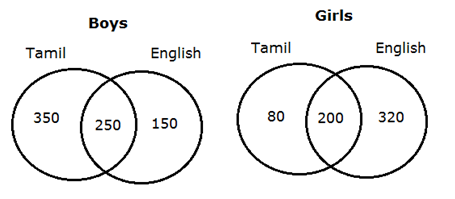# RBI Assistant Prelims Quantitative Aptitude Questions 2019-20 – (Day-02)

Dear Aspirants, Our IBPS Guide team is providing new series of Quantitative Aptitude Questions for RBI Assistant Prelims 2019-20 so the aspirants can practice it on a daily basis. These questions are framed by our skilled experts after understanding your needs thoroughly. Aspirants can practice these new series questions daily to familiarize with the exact exam pattern and make your preparation effective.

Ensure Your Ability Before of Exam

Take RBI Assistant Prelims Free Mock test

[WpProQuiz 7556]

Missing number series

Directions (01 – 05): What value should come in the place of (?) in the following number series?

1) 1, 145, 266, ?, 447, 511

a) 286

b) 296

c) 336

d) 366

e) 378

2) 19, 40, 82, 166, 334, ?

a) 429

b) 450

c) 670

d) 680

e) 720

3) 14, 24, 36, 50, 66, ?

a) 70

b) 72

c) 78

d) 80

e) 84

4) 13, 37, 65, 97, 133, ?

a) 192

b) 189

c) 173

d) 166

e) 150

5) 9, 30, 74, 143, 239, ?

a) 364

b) 376

c) 388

d) 399

e) 339

Caselet

Directions (6 – 10): Study the following information carefully and answer the questions given below.

A school has the number of boys to girls in the ratio of 5: 4 and the each of them like at least one of two languages Tamil and English. One – third of the student likes both Tamil and English. 280 girls like Tamil. 33.33% of the boys like both Tamil and English. One – fifth of the boys like only English and 8/15th of the girls like only English.

6) What is the difference between the number of girls like only English and the number of boys like only Tamil?

a) 120

b) 150

c) 170

d) 190

e) 180

7) The number of girls like both languages is what percent of the total number of boys like Tamil?

a) 30%

b) 40%

c) 50%

d) 60%

e) None of these

8) Find the total number of students in the school.

a) 2000

b) 2200

c) 2400

d) 1500

e) None of these

9) What is the ratio of the number of boys like English to the number of girls like English?

a) 15: 11

b) 11: 7

c) 17: 13

d) 15: 13

e) None of these

10) The number of boys only like English is approximately what percent of the total number of girls in the school?

a) 58%

b) 46%

c) 48%

d) 50%

e) 52%

Directions (1-5) :

1 + 122 = 145

145 + 112 = 266

266 + 102 = 366

366 + 92 = 447

447 + 82 = 511

19 * 2 + 2 = 40

40 * 2 + 2 = 82

82 * 2 + 2 = 166

166 * 2 + 2 = 334

334 * 2 + 2 = 670

22 + 10 = 14

32 + 15 = 24

42 + 20 = 36

52 + 25 = 50

62 + 30 = 66

72 + 35 = 84

13 + 24 = 37

37 + 28 = 65

65 + 32 = 97

97 + 36 = 133

133 + 40 = 173

9 + (21 * 1) = 30

30 + (22 * 2) = 74

74 + (23 * 3) = 143

143 + (24 * 4) = 239

239 + (25 * 5) = 364

Directions (6 – 10):

Total number of students = 9x

Total number of girls = 4x

Total number of boys = 5x

Total girls like only English = 8/15th of the total girls

Total girls like only English to total girls = 8: 15

Number of girls like Tamil = 15y – 8y = 7y = 280

= > y = 280/7 = 40

Total girls like only English = 8 * 40 = 320

Total girls = 40 * 15 = 600

Total boys = 600/4 * 5 = 750

Total students like both Tamil and English = (600 + 750)/3 = 450

Total boys like both Tamil and English = 33.33% of 750 = 250

Total girls like both Tamil and English = 450 – 250 = 200

Total girls like Only Tamil = 280 – 200 = 80

Total boys like only English = 750 * 1/5 = 150

Total boys like only Tamil = 750 – (250 + 150)

= 750 – 400 = 350Difference = 320 – 150 = 170

Required percentage = (200/400) * 100 = 50%

Total students in the school = 750 + 600 = 1350

Required ratio = 600: 520

= 15: 13